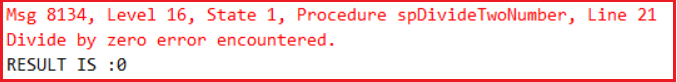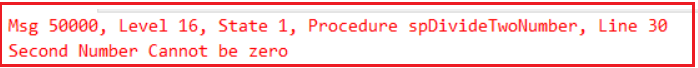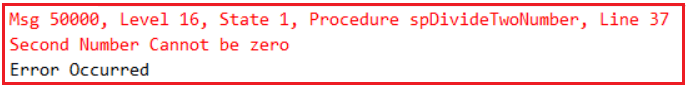# Exception Handling in SQL Server

## Exception Handling in SQL Server with Examples

In this article, I am going to discuss Exception Handling in SQL Server with Examples. As a developer, it is very important for you to understand Exception Handling in SQL Server. As part of this article, we are going to discuss the following pointers related to SQL Server Exception Handling.

1. Why do we need Exception Handling in SQL Server?
2. What Happens in SQL Server when an Exception Occurred?
3. What is Exception handling in SQL Server?
4. Exception Handling Using RAISERROR System Function.
5. Understanding the RaiseError System Function in SQL Server.
6. Using @@Error System Function in SQL Server
7. Understanding Error Number, Error Message, Severity Level, and Error State
##### Why do we need Exception Handling in SQL Server?

Let us understand the need for exception handling in SQL Server with an example. So, create a SQL Server Stored Procedure for dividing two numbers by executing the below query.’

```CREATE PROCEDURE spDivideTwoNumber(
@Number1 INT,
@Number2 INT
)
AS
BEGIN
DECLARE @Result INT
SET @Result = 0
SET @Result = @Number1 / @Number2
PRINT 'RESULT IS :'+CAST(@Result AS VARCHAR)
END```

Execution: Now call the stored procedure by giving the numbers as 100 and 5 as shown below
EXEC spDivideTwoNumber 100, 5
When you execute the above, you will get the following output
RESULT IS: 20

Now execute the stored procedure by giving the second value as 0 as shown below.
EXEC spDivideTwoNumber 100, 0
When we execute the procedure with 100 and 0, we get the following error. This is because the divisor value is 0 as we know a number cannot be divided by zero as per the rule of mathematics.As you can see in the above image, whenever an error occurs at a particular line, in SQL Server, it will first print that error message and then it will continue with its execution. So in our example, the statement SET @Result = @Number1 / @Number2 is the error statement in the procedure and once this statement executes with the second number as 0, it will print Divide by zero error message and then continues its execution with from next statement onwards and this is the reason why it still prints the message “RESULT IS :0”.

So, the problem with the above execution is that even if an error occurred in the program, it still showing the result so there are chances of users being confused.

##### What Happens in SQL Server when an Exception Occurred?

In SQL Server, whenever an exception occurred, it displays the exception message and then continues the program execution. But in programming languages such as C#, Java, C++, etc. whenever an exception occurred then the program execution terminates abnormally on the line where the exception occurred.

In the above case both the behaviors are wrong because when errors occur in a programming language it will skip the execution of all the statements after the error even if those statements are not related to the error whereas in SQL Server the execution will not stop when the error occurred, statements related with the error also executed but it should not be happening. For example in the above-stored procedure when the exception occurred it is still displaying the “RESULT IS: 0” which should not happen.

##### What is Exception handling in SQL Server?

We handle errors both in programming languages as well as in databases. Handling errors in a programming language means stopping the abnormal termination of the program and allowing the statements which are not related to the error to execute but handling an error in SQL Server means stopping the execution of the statements which are related to the error.

With the introduction of Try/Catch blocks in SQL Server 2005, the error handling in the SQL server is now very much similar to programming languages like C#, and Java. But, before understanding the error handling using the try/catch block, let’s step back and understand how error handling was done in SQL Server prior to 2005, using the system function RAISERROR and @@Error.

##### Exception Handling Using RAISERROR System Function in SQL Server:

Let’s alter the same stored procedure that we created in the previous example as shown below to use the Raiseerror System Function to handling the exception in SQL Server.

```ALTER PROCEDURE spDivideTwoNumber
@Number1 INT,
@Number2 INT
AS
BEGIN
DECLARE @Result INT
SET @Result = 0
IF(@Number2 = 0)
BEGIN
RAISERROR('Second Number Cannot be zero', 16, 1)
END
ELSE
BEGIN
SET @Result = @Number1 / @Number2
PRINT 'RESULT IS : '+ CAST(@Result AS VARCHAR)
END
END```

Let’s execute the procedure by giving the second value as zero as shown below.
EXEC spDivideTwoNumber 100, 0
When we execute the above procedure with 100 and 0, it gives us the below error message.In the above procedure, we are using the system-defined Raiserror() function to return an error message back to the calling application, if the second number is zero.

##### What is RaiseError System Function in SQL Server?

The RaiseError System defined Function in SQL Server takes 3 parameters as shown below.
RAISERROR(‘Error Message’, ErrorSeverity, ErrorState)

1. Error Message: The custom error message that you want to display whenever the exception is raised.
2. Error Severity: When we are returning any custom errors in SQL Server, we need to set the ErrorSeverity level as 16, which indicates this is a general error and this error can be corrected by the user. In our example, the error can be corrected by the user by giving a nonzero value for the second parameter.
3. Error State: The ErrorState is also an integer value between 1 and 255. The RAISERROR() function can only generate custom errors if you set the Error State value between 1 to 127.
##### @@Error System Function in SQL Server:

In SQL Server 2000, in order to detect errors, we use the @@Error system function. The @@Error system function returns a NON-ZERO value if there is an error, otherwise, ZERO indicates that the previous SQL statement was executed without any error. Let’s modify the stored procedure to make use of the @@ERROR system function as shown below.

```ALTER PROCEDURE spDivideTwoNumber
@Number1 INT,
@Number2 INT
AS
BEGIN
DECLARE @Result INT
SET @Result = 0
IF(@Number2 = 0)
BEGIN
RAISERROR('Second Number Cannot be zero',16,1)
END
ELSE
BEGIN
SET @Result = @Number1 / @Number2
END

IF(@@ERROR <> 0)
BEGIN
PRINT 'Error Occurred'
END
ELSE
BEGIN
PRINT 'RESULT IS :'+CAST(@Result AS VARCHAR)
END
END```

Let’s execute the procedure as EXEC spDivideTwoNumber 100, 0
When we execute the above procedure passing 100 and 0, it gives us the below output.##### Predefined Error Terms in SQL Server:

Whenever an error occurs under a program like dividing a number by zero, violation of primary key, violation of Check constraint, etc. the system displays an error message telling us the problem encountered in the code. Every error that occurs in the program is associated with four attributes.

1. Error Number
2. Error Message
3. Severity Level
4. Error State
##### Example:

Message 8134 (Error Number), Level 16(SEVERITY Level), State 1 (state), Divide by Zero error encountered (Error Message)

Error Number: The Error number is a unique identifier given for each and every error that occurs in SQL Server. This value will be below 50,000 for predefined errors and must be above or equals to 50,000 for errors defined by the user. While raising custom errors, if we don’t specify the error number, then by default it will set the Error Number as 50000.

Error Message: It is a piece of brief information describing the error that occurred which should be maxing from 2047 characters.

Severity Level: This tells about the importance of the error which can be ranging between 0 to 24. In which

1. 0 to 9: are not serves which can be considered as information or status messages.
2. 11 to 16:  Indicates these errors can be created by the user.
3. 17 to 19: Indicates these are software errors that cannot be corrected by the user must be reported to the system administrator.
4. 20 to 24: Indicates fatal errors and if these errors occur they can damage the system or database. So here the connection immediately terminates with the database.

State: It is an arbitrary value that is not that important can be ranging between 0 to 127. We use this whenever the same error has to occur in multiple places.

Note: We can find the information of all predefined errors under the table “Sys Messages

In the next article, I am going to discuss the RaiseError and @@ERROR in SQL Server with Examples. Here, in this article, I try to explain the basics of Exception Handling in SQL Server with examples. I hope you enjoy this Exception Handling in the SQL Server article.